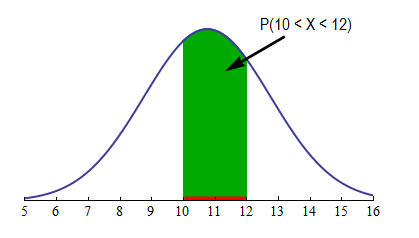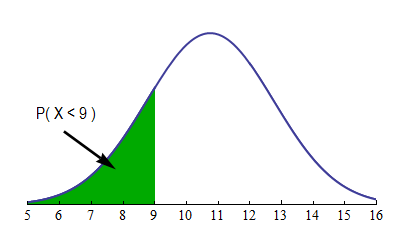## Continuous Probability Distribution (2 of 2)

### Learning Objectives

• Use a probability distribution for a continuous random variable to estimate probabilities and identify unusual events.

Previously, we examined the probability distribution for foot length. For foot length and for all other continuous random variables, the probability distribution can be approximated by a smooth curve called a probability density curve.

Recall that these smooth curves are mathematical models. We use a mathematical model to describe a probability distribution so that we can use technology and the equation of this model to estimate probabilities. (As we mentioned earlier, we do not study the equation for this curve in this course, but every statistical package uses this equation, and the area under the corresponding curve, to estimate probabilities.)As in a probability histogram, the total area under the density curve equals 1, and the curve represents probabilities by area. To find the probability that X is in an interval, find the area above the interval and below the density curve.

For example, if X is foot length, let’s find P(10 < X < 12), the probability that a randomly chosen male has a foot length anywhere between 10 and 12 inches. This probability is the area above the interval 10 < X < 12 and below the curve. We shaded this area with green in the following graph.If, for example, we are interested in P(X < 9), the probability that a randomly chosen male has a foot length of less than 9 inches, we have to find the area shaded in green below: